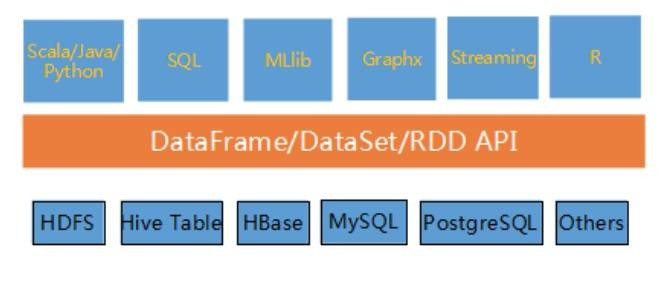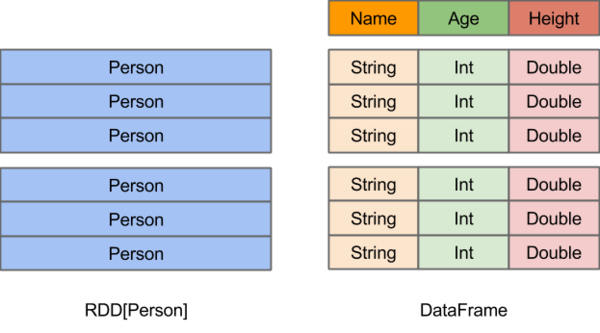Spark Core组件:RDD、DataFrame和DataSet

0
0
01. 云栖社区>
2. 博客>
3. 正文

Spark Core组件:RDD、DataFrame和DataSet

wsc449 2018-01-17 09:11:26 浏览727

1. 介绍

spark生态系统中，Spark Core，包括各种Spark的各种核心组件，它们能够对内存和硬盘进行操作，或者调用CPU进行计算。
spark core定义了RDD、DataFrame和DataSetspark最初只有RDD，DataFrame在Spark 1.3中被首次发布，DataSet在Spark1.6版本中被加入。

2. RDD

RDD：Spark的核心概念是RDD (resilientdistributed dataset)，指的是一个只读的，可分区的分布式数据集，这个数据集的全部或部分可以缓存在内存中，在多次计算间重用。

GC的性能开销

import org.apache.spark.sql.SQLContext
import org.apache.spark.{SparkConf, SparkContext}

object Run {
def main(args: Array[String]) {
val conf = new SparkConf().setAppName("test").setMaster("local")
val sc = new SparkContext(conf)
sc.setLogLevel("WARN")
val sqlContext = new SQLContext(sc)

/**
* id      age
* 1       30
* 2       29
* 3       21
*/
case class Person(id: Int, age: Int)
val idAgeRDDPerson = sc.parallelize(Array(Person(1, 30), Person(2, 29), Person(3, 21)))

// 优点1
// idAge.filter(_.age > "") // 编译时报错, int不能跟String比

// 优点2
idAgeRDDPerson.filter(_.age > 25) // 直接操作一个个的person对象
}
}

3. DataFrameDataFrame引入了schema和off-heap

schema : RDD每一行的数据, 结构都是一样的.

off-heap就像地盘，schema就像地图， Spark有地图又有自己地盘了， 就可以自己说了算了， 不再受JVM的限制，也就不再收GC的困扰了。通过schema和off-heap，DataFrame解决了RDD的缺点，但是却丢了RDD的优点。 DataFrame不是类型安全的， API也不是面向对象风格的。

import org.apache.spark.sql.types.{DataTypes, StructField, StructType}
import org.apache.spark.sql.{Row, SQLContext}
import org.apache.spark.{SparkConf, SparkContext}

object Run {
def main(args: Array[String]) {
val conf = new SparkConf().setAppName("test").setMaster("local")
val sc = new SparkContext(conf)
sc.setLogLevel("WARN")
val sqlContext = new SQLContext(sc)
/**
* id      age
* 1       30
* 2       29
* 3       21
*/
val idAgeRDDRow = sc.parallelize(Array(Row(1, 30), Row(2, 29), Row(4, 21)))

val schema = StructType(Array(StructField("id", DataTypes.IntegerType), StructField("age", DataTypes.IntegerType)))

val idAgeDF = sqlContext.createDataFrame(idAgeRDDRow, schema)
// API不是面向对象的
idAgeDF.filter(idAgeDF.col("age") > 25)
// 不会报错, DataFrame不是编译时类型安全的
idAgeDF.filter(idAgeDF.col("age") > "")
}
}

4. DataSet

Dataset是一个强类型的特定领域的对象，这种对象可以函数式或者关系操作并行地转换。每个Dataset也有一个被称为一个DataFrame的类型化视图，这种DataFrame是Row类型的Dataset，即Dataset[Row]

Dataset是“懒惰”的，只在执行行动操作时触发计算。本质上，数据集表示一个逻辑计划，该计划描述了产生数据所需的计算。当执行行动操作时，Spark的查询优化程序优化逻辑计划，并生成一个高效的并行和分布式物理计划。

DataSet结合了RDD和DataFrame的优点,，并带来的一个新的概念Encoder 当序列化数据时，Encoder产生字节码与off-heap进行交互，能够达到按需访问数据的效果， 而不用反序列化整个对象。 Spark还没有提供自定义Encoder的API，但是未来会加入。

// 上文DataFrame示例中提取出来的
val idAgeRDDRow = sc.parallelize(Array(Row(1, 30), Row(2, 29), Row(4, 21)))

val schema = StructType(Array(StructField("id", DataTypes.IntegerType), StructField("age", DataTypes.IntegerType)))

val idAgeDF = sqlContext.createDataFrame(idAgeRDDRow, schema)

// sqlContext.createDataFrame(idAgeRDDRow, schema) 方法的实现, 返回值依然是DataFrame
def createDataFrame(rowRDD: RDD[Row], schema: StructType): DataFrame = {
sparkSession.createDataFrame(rowRDD, schema)
}

package object sql {
// ...省略了不相关的代码

type DataFrame = Dataset[Row]
}

import org.apache.spark.sql.types.{DataTypes, StructField, StructType}
import org.apache.spark.sql.{Row, SQLContext}
import org.apache.spark.{SparkConf, SparkContext}

object Test {
def main(args: Array[String]) {
val conf = new SparkConf().setAppName("test").setMaster("local") // 调试的时候一定不要用local[*]
val sc = new SparkContext(conf)
val sqlContext = new SQLContext(sc)
import sqlContext.implicits._

val idAgeRDDRow = sc.parallelize(Array(Row(1, 30), Row(2, 29), Row(4, 21)))

val schema = StructType(Array(StructField("id", DataTypes.IntegerType), StructField("age", DataTypes.IntegerType)))

// 在2.0.0-preview中这行代码创建出的DataFrame, 其实是DataSet[Row]
val idAgeDS = sqlContext.createDataFrame(idAgeRDDRow, schema)

// 在2.0.0-preview中, 还不支持自定的Encoder, Row类型不行, 自定义的bean也不行
// 官方文档也有写通过bean创建Dataset的例子，但是我运行时并不能成功
// 所以目前需要用创建DataFrame的方法, 来创建DataSet[Row]
// sqlContext.createDataset(idAgeRDDRow)

// 目前支持String, Integer, Long等类型直接创建Dataset
Seq(1, 2, 3).toDS().show()
sqlContext.createDataset(sc.parallelize(Array(1, 2, 3))).show()
}
}

5. RDD和DataFrame比较DataFrame与RDD相同之处，都是不可变分布式弹性数据集。不同之处在于，DataFrame的数据集都是按指定列存储，即结构化数据。类似于传统数据库中的表。
DataFrame的设计是为了让大数据处理起来更容易。DataFrame允许开发者把结构化数据集导入DataFrame，并做了higher-level的抽象; DataFrame提供特定领域的语言（DSL）API来操作你的数据集。

6. RDD和DataSet比较

DataSet以Catalyst逻辑执行计划表示，并且数据以编码的二进制形式被存储，不需要反序列化就可以执行sorting、shuffle等操作。

DataSet创立需要一个显式的Encoder，把对象序列化为二进制，可以把对象的scheme映射为Spark SQl类型，然而RDD依赖于运行时反射机制。

7. DataFrame和DataSet比较

Dataset可以认为是DataFrame的一个特例，主要区别是Dataset每一个record存储的是一个强类型值而不是一个Row。因此具有如下三个特点：
1.DataSet可以在编译时检查类型
2.是面向对象的编程接口。用wordcount举例：
3.后面版本DataFrame会继承DataSet，DataFrame是面向Spark SQL的接口。
DataFrame和DataSet可以相互转化，df.as[ElementType]这样可以把DataFrame转化为DataSet，ds.toDF()这样可以把DataSet转化为DataFrame。
//DataFrame

// Load a text file and interpret each line as a java.lang.String
val ds = sqlContext.read.text("/home/spark/1.6/lines").as[String]
val result = ds
.flatMap(_.split(" "))               // Split on whitespace
.filter(_ != "")                     // Filter empty words
.toDF()                              // Convert to DataFrame to perform aggregation / sorting
.groupBy(\$"value")                   // Count number of occurences of each word
.agg(count("*") as "numOccurances")
.orderBy(\$"numOccurances" desc)      // Show most common words first

//DataSet,完全使用scala编程，不要切换到DataFrame

val wordCount =
ds.flatMap(_.split(" "))
.filter(_ != "")
.groupBy(_.toLowerCase()) // Instead of grouping on a column expression (i.e. \$"value") we pass a lambda function
.count()

8. 应用场景

RDD在Apache Spark 2.0中惨遭抛弃？

// select specific fields from the Dataset, apply a predicate
// using the where() method, convert to an RDD, and show first 10
// RDD rows

val deviceEventsDS = ds.select(\$"device_name", \$"cca3", \$"c02_level").where(\$"c02_level" > 1300)
// convert to RDDs and take the first 10 rows

val eventsRDD = deviceEventsDS.rdd.take(10)

wsc449
+ 关注

corcosa 15376人浏览